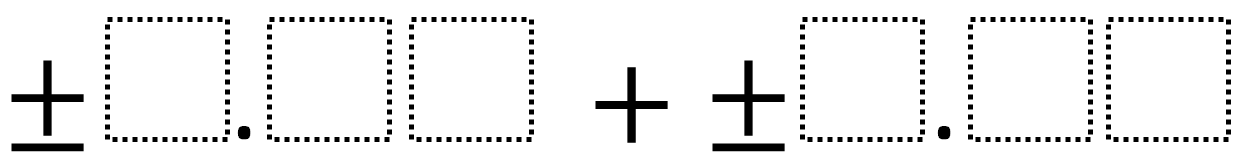Directions: Using the digits 1 to 9 at most one time each, fill in the boxes to make the smallest (or largest) sum.### Hint

What number does each box represent?

No answers yet.  If you have one, post it in the comments.

Note: This problem’s difficulty can be adjusted by altering the number of digits (boxes), picking smallest or largest, or by picking either a positive, negative, or both.

Source: Robert Kaplinsky

## Solving One-Step Inequalities with Addition

Directions: Using the digits 0 to 9 at most one time each, place a digit …

1.The smallest number you can make is 3.81. I know this because your top six smallest numbers you have to use to get the smallest sum. Your top six smallest numbers, 1-9, are 1, 2, 3, 4, 5, and 6. The two smallest numbers go in the greatest column [whole number], the next two smallest go in the next greatest column [tenths], and your last two numbers go in the least greatest column [hundredths]. When you place the numbers in the correct columns, your two numbers will be 1.35 and 2.46. When you add the columns together, you have your sum of 3.81.

2.Hi Robert!

I played with this one recently with some teachers. We came up with a few variations we thought strived at getting at the place value concept a bit more deeply. (We only looked at positive decimals.)

We came up with something like: ___ ___ . ___ ___ + 0 . ___ ___ ___

And: ___ ___ ___ + ___ . ___ ___ + ___ ___ . ___

We were trying to think of ways to nudge students out of auto-pilot (those who were just putting the largest number in the leftmost space without conceptually understanding why). Not sure if those are worth adding in or not, but wanted to help support this rad project!

Cheers!
co

3.For __ . __ __ + (- __ . __ __) AKA __ . __ __ – __ . __ __ students came up with 1.23-9.87= -8.64 .

4.5.The smallest sum is obtained by using the negative signs in both numbers, and their largest absolute value: -9.75 + -8.64 = -18.39
There are different arrangements of digits that give the same sum, just use the largest digits in the largest place values.

6.largest
9.75+8.64=18.39

7.largest
9.75+8.64=18.39

8.1.23+4.56=5.79 thats the small

9.1.23+4.56=5.79 thats the smallest answer i got

10.1.23+4.56=5.79 that is the smallest ansawer weho i got it